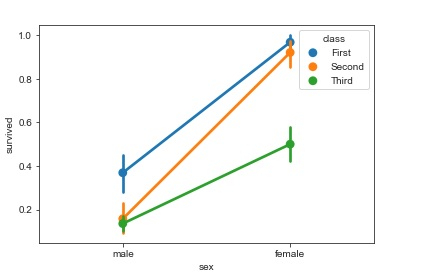# How can Seaborn library be used to visualize point plots in Python?

Seaborn is a library that helps in visualizing data. This interface helps in customizing and controlling the kind of data and how it behaves when certain filters are applied to it.

With the help of bar plots, we can understand the central tendency of the distribution of data. The barplot function establishes the relationship between a categorical variable and a continuous variable.

Data is represented in the form of rectangular bars where the length of the bar indicates the proportion of data in that specific category.

Point plots are similar to bar plots but instead of representing the fill bar, the estimated value of the data point is represented by a point at a specific height on the other axis. Here’s the example −

## Example

import pandas as pd
import seaborn as sb
from matplotlib import pyplot as plt
sb.pointplot(x = "sex", y = "survived", hue = "class", data = my_df)
plt.show()

## Output## Explanation

• The required packages are imported.
• The input data is ‘titanic’ which is loaded from the seaborn library.
• This data is stored in a dataframe.